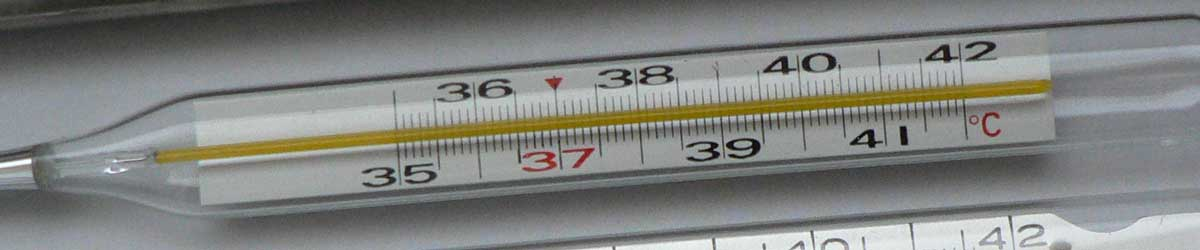# Celsius to Rankine Conversion

Enter the temperature in Celsius below to get the value converted to Rankine.

Results in Degrees Rankine:20 °C = 527.67 °R
Do you want to convert Rankine to Celsius?

## How to Convert Celsius to RankineConvert degrees Celsius to degrees Rankine with this simple formula:

degrees Rankine = ( [°C] + 273.15 ) × 9 / 5

Insert the [°C] temperature measurement in the formula and then solve to find the result.

For example, let's convert 50 °C to degrees Rankine:

50 °C = ( ( 50 + 273.15 ) × 9 / 5 ) = 581.67 °R

## Celsius

The Celsius temperature scale, also commonly referred to as the centigrade scale, is defined in relation to the kelvin. Specifically, degrees Celsius is equal to kelvins minus 273.15.

The degree Celsius is the SI derived unit for temperature in the metric system. A degree Celsius is sometimes also referred to as a degree centigrade. Celsius can be abbreviated as C; for example, 1 degree Celsius can be written as 1 °C.

### Important Temperatures In Degrees Celsius

TemperatureDegrees Celsius
Absolute Zero-273.15 °C
Freezing Point of Water0 °C
Triple Point of Water0.01 °C
Boiling Point of Water100 °C

## Rankine

The Rankine scale is an absolute temperature scale that uses absolute zero as the starting point, much like the Kelvin scale. The key difference between the Rankine scale and the Kelvin scale is that an increment of one degree rankine is equal to an increment of one degree Fahrenheit, not Celsius as in the Kelvin scale. The base of the Rankine scale starts at absolute zero, thus 0 degrees rankine are equal to −459.67 degrees Fahrenheit.

Rankine can be abbreviated as R, and are also sometimes abbreviated as Ra. For example, 1 degree Rankine can be written as 1 °R or 1 Ra.

### Important Temperatures In Degrees Rankine

TemperatureDegrees Rankine
Absolute Zero0 °R
Freezing Point of Water491.67 °R
Triple Point of Water491.688 °R
Boiling Point of Water671.67 °R

## Celsius to Rankine Conversion Table

Celsius measurements converted to Rankine
Celsius Rankine
-273.15 °C 0 °R
-50 °C 401.67 °R
-40 °C 419.67 °R
-30 °C 437.67 °R
-20 °C 455.67 °R
-10 °C 473.67 °R
0 °C 491.67 °R
10 °C 509.67 °R
20 °C 527.67 °R
30 °C 545.67 °R
40 °C 563.67 °R
50 °C 581.67 °R
60 °C 599.67 °R
70 °C 617.67 °R
80 °C 635.67 °R
90 °C 653.67 °R
100 °C 671.67 °R
110 °C 689.67 °R
120 °C 707.67 °R
130 °C 725.67 °R
140 °C 743.67 °R
150 °C 761.67 °R
160 °C 779.67 °R
170 °C 797.67 °R
180 °C 815.67 °R
190 °C 833.67 °R
200 °C 851.67 °R
300 °C 1,032 °R
400 °C 1,212 °R
500 °C 1,392 °R
600 °C 1,572 °R
700 °C 1,752 °R
800 °C 1,932 °R
900 °C 2,112 °R
1,000 °C 2,292 °R

## References

1. National Institute of Standards and Technology, SI Units - Temperature, https://www.nist.gov/pml/weights-and-measures/si-units-temperature